### Home > A2C > Chapter 12 > Lesson 12.3.2 > Problem12-149

12-149.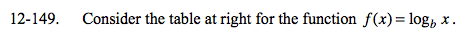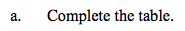Use the logarithm rules to calculate the missing values.

logb 4 = logb (22) = 2(logb 2)
logb 4 = 0.5404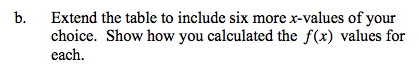See part (a).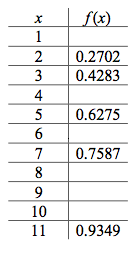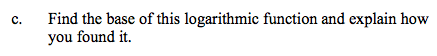When does logb x = 1? If you cannot find this,
when does logb x become greater than one?